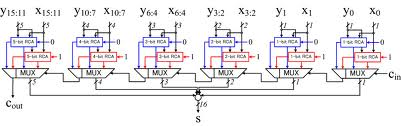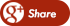﻿ DESIGN AND PERFORMANCE ANALYSIS OF VARIOUS ADDERSBack

## DESIGN AND PERFORMANCE ANALYSIS OF VARIOUS ADDERSThe designs of various adders are  as follows:

• Its an basic adder which  works on basic logic principle.
• The two exclusive-OR gate performe an sum of two single bit addition.
• And three  AND gate and one OR gate  perform the carry for two single bit.
• The combination of these function is known as FULL ADDER(FA)  unit.
• These FA unit can connect serially to achive number of addition.
• The carry can ripple through each FA unit to produce the final arithmetic results.
•  So the delay is more as the number of bits increases.
• Hence its known Riiple Carry Adder.
• AREA (LUT)=8;
• DELAY(ns) =2.191.• This adder is designed to speed up arithmetic operation by adding the propagation of carry bit.
• The carry skip circuitry consists of AND gate accept the carry-in bit and compare with group of  propagted signals.
• It uses skip logic in propagation of carry.
• The delay is high compared with RCA.
• This is one of  the efficient adder design
• Hence its known as Carry Skip Adder.
• AREA (LUT)=8;
• DELAY(ns) =2.296.• This adder is designed with the combination of Ripple Carry Adder and incrementation circuitry.
• The incrementation circuitry is design using Half Adder in Ripple Carry Chain in a sequential order.
• The Addition operation is done by dividing the total  number of bits into number of groups
• This is one of the  efficient adder design.
• Delay is less compared with RCA
• AREA (LUT)=8.
• DELAY(ns) =1.907.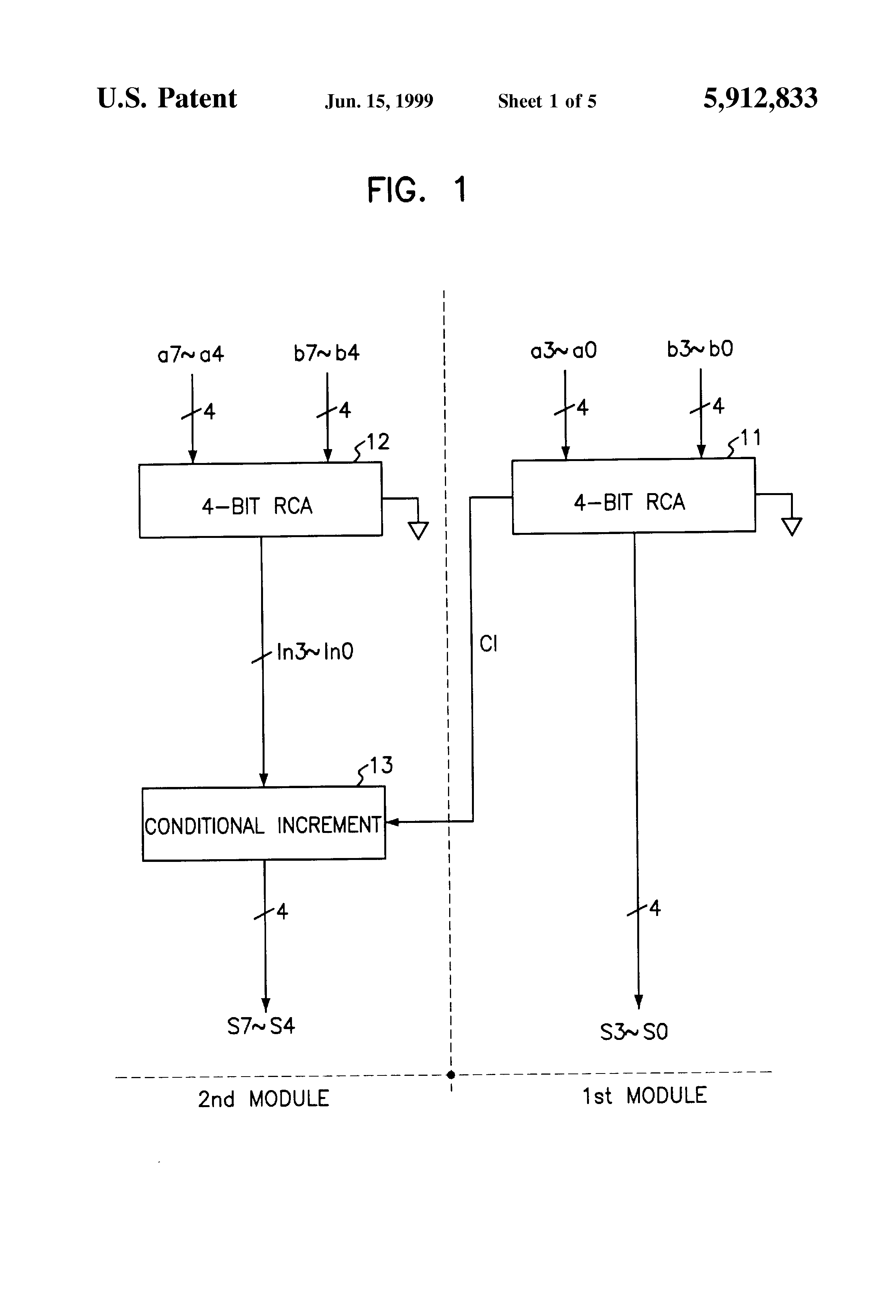• This  adder is design with the Ripple Carry Adder  and By-pass circuitry.
• During number of bit addition carry generated will be propagated to next stage with help of multiplexer using select input as By-pass logic.
• Depending on the carry value and By-pass logic, the carry is propagated to the next FA unit.
• By-pass logic is formed from the product values as it is calculated in the CLA.
• Delay is high compared with RCA adder.
• AREA(LUT) =12.
• DELAY(ns)  =3.160.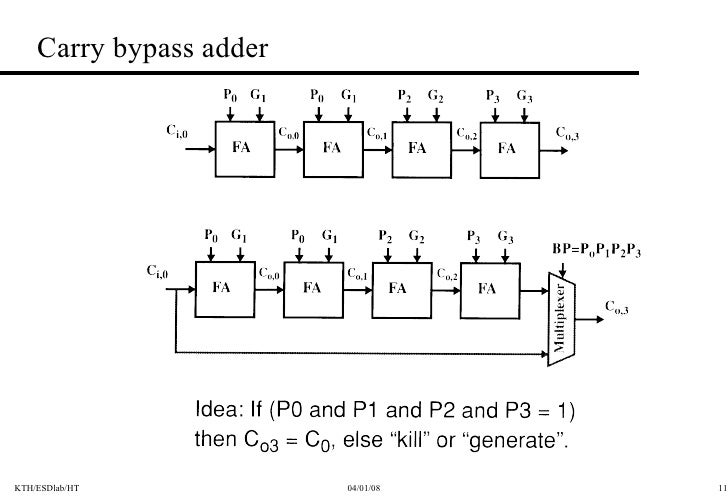• This adder design is based on the principle of looking at lower adder bits of argument and addend if higher orders carry generated.
• This adder reduces the carry delay by reducing the number of gates through which a carry signal must propagate.
• The generation and propagation stage,the generation values, propagation values are computed.
• Internal carry generation is calculated in second stage. and in final stage, the sum is calculated.
• AREA(LUT) =10.
• DELAY(ns) =2.266.• The number of bits are added parallely at same time.
• The carry is not propagated through the stages and the carry is stored in present stage.
• Hence, the delay due to the carry is reduced.
• AREA (LUT)=13.
• DELAY(ns) =1.433.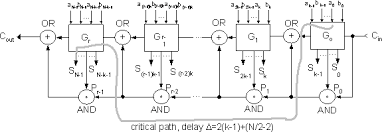• The Architecture consists of independent generation of sum and carry i.e., Cin=1
and Cin=0 are executed parallelly
• Depending upon Cin, the external multiplexers select the carry to be propagated.
• Based on the carry input, the sum will be selected.
• Hence, the delay is reduced.
• However, the structure is increased due to the complexity of multiplexers.
• AREA(LUT) =8.
• DELAY(ns) =2.588.